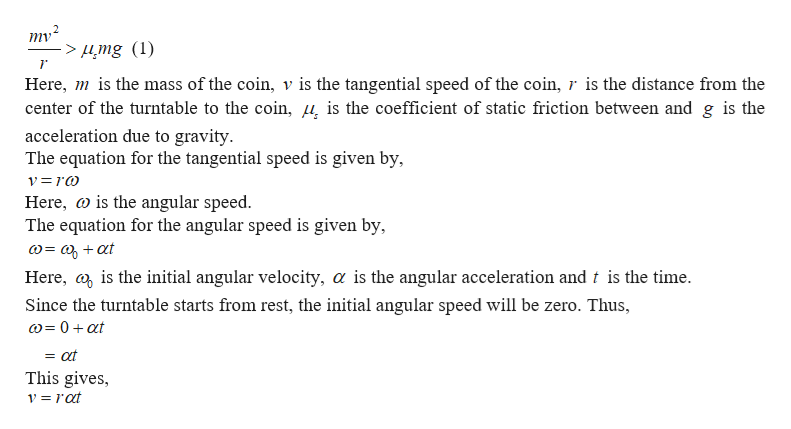# A coin rests 15.0 cm from the center of a turntable. Thecoefficient of static friction between the coin and turntablesurface is 0.350. The turntable starts from rest at t = 0 androtates with a constant angular acceleration of 0.730 rad/s2.(a) Once the turntable starts to rotate, what force causes thecentripetal acceleration when the coin is stationary relativeto the turntable? Under what conditiondoes the coin begin to move relativeto the turntable?(b) After whatperiod of time will the coin start toslip on the turntable?

Question
40 views

A coin rests 15.0 cm from the center of a turntable. The
coefficient of static friction between the coin and turntable
surface is 0.350. The turntable starts from rest at t = 0 and
rotates with a constant angular acceleration of 0.730 rad/s2.

(a) Once the turntable starts to rotate, what force causes the
centripetal acceleration when the coin is stationary relative
to the turntable? Under what condition
does the coin begin to move relative
to the turntable?

(b) After what
period of time will the coin start to
slip on the turntable?

check_circle

Step 1

(a)If there is no friction between the surface of the turntable and the coin, the coin will go away from the center. To overcome this motion, the static frictional force must act towards the center of the turntable. When the coin begins...help_outlineImage Transcriptionclosemy? > µmg (1) Here, m is the mass of the coin, v is the tangential speed of the coin, r is the distance from the center of the turntable to the coin, µ̟ is the coefficient of static friction between and g is the acceleration due to gravity. The equation for the tangential speed is given by, v=ro Here, w is the angular speed. The equation for the angular speed is given by, @= a + at Here, a, is the initial angular velocity, a is the angular acceleration and t is the time. Since the turntable starts from rest, the initial angular speed will be zero. Thus, w= 0+ at = at This gives, v = rat fullscreen

### Want to see the full answer?

See Solution

#### Want to see this answer and more?

Solutions are written by subject experts who are available 24/7. Questions are typically answered within 1 hour.*

See Solution
*Response times may vary by subject and question.
Tagged in

### Kinematics